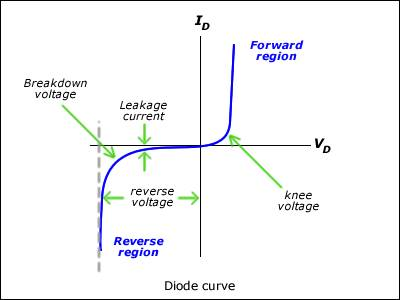Diode Equation and Saturation Current

Good day all:

For the diode equation: $$I(V)=I_{sat}e^{\frac{Ve}{nKT}}-I_{sat}$$

I also know that the second term in the equation is negligible if: $$V> > nV_{T}$$

Vt is thermal voltage
n is ....just a factor related to the quality of the material

I have these initial conditions:
$$V> 0.1V\, \, \, ,kT\approx .025 eV\, \, \, n\approx 2$$

Its easy to show that the my inital conditions lead to a V much greater that n*thermal voltage.

My question is: Why is the saturation Current negligible? I know it has something to do with recombination rate but can anyone give me a simple explanation? So many websites just say that the order relation is true but give no evidence as to why.